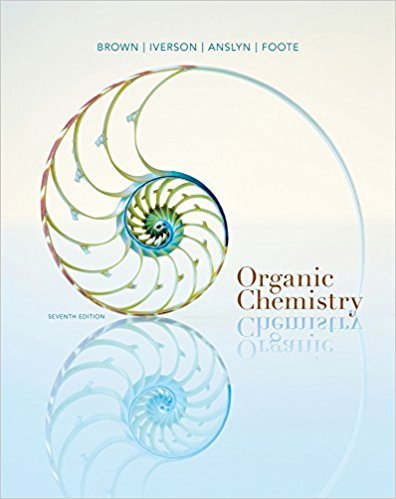×
Get Full Access to Organic Chemistry - 7 Edition - Chapter 13 - Problem 13.16
Get Full Access to Organic Chemistry - 7 Edition - Chapter 13 - Problem 13.16

×

# Following are 1H-NMR spectra for compounds D, E, and F, each with molecularISBN: 9781133952848 483

## Solution for problem 13.16 Chapter 13

Organic Chemistry | 7th Edition

• Textbook Solutions
• 2901 Step-by-step solutions solved by professors and subject experts
• Get 24/7 help from StudySoup virtual teaching assistantsOrganic Chemistry | 7th Edition

4 5 1 374 Reviews
15
4
Problem 13.16

Following are 1H-NMR spectra for compounds D, E, and F, each with molecular formulaC6H12. Each readily decolorizes a solution of Br2 in CCl4. Propose structural formulas forcompounds D, E, and F and account for the observed patterns of signal splitting.

Step-by-Step Solution:
Step 1 of 3

1. Matter can change either physically or chemically. 1. In our body systems, we often use special chemicals called enzymes to help chemically change matter. Elements A. Elements are the building blocks of chemical matter. These are substances that cannot be broken down into simpler substances by ordinary chemical reactions. B. There are more than 100 elements. Most elements are naturally occurring, but there are some man-made elements. Elements are given names and abbreviations (chemical symbols) indicated by letters.

Step 2 of 3

Step 3 of 3

## Discover and learn what students are asking

Calculus: Early Transcendental Functions : Conics, Parametric Equations, and Polar Coordinates
?In Exercises 1–6, match the equation with the correct graph. [The graphs are labeled (a), (b), (c), (d), (e), and (f).]

Calculus: Early Transcendental Functions : Multiple Integration
?In Exercises 1 and 2, evaluate the integral. $$\int_{0}^{2 x} x y^{3} d y$$

Statistics: Informed Decisions Using Data : Introduction to the Practice of Statistics
?A(n) ________ is a numerical summary of a sample. A(n) ________ is a numerical summary of a population.

Statistics: Informed Decisions Using Data : Comparing Three or More Means (One-Way Analysis of Variance)
?Car-Buying Discrimination To determine if there is gender and/or race discrimination in car buying, Ian Ayres put together a team of fifteen white mal

Unlock Textbook Solution